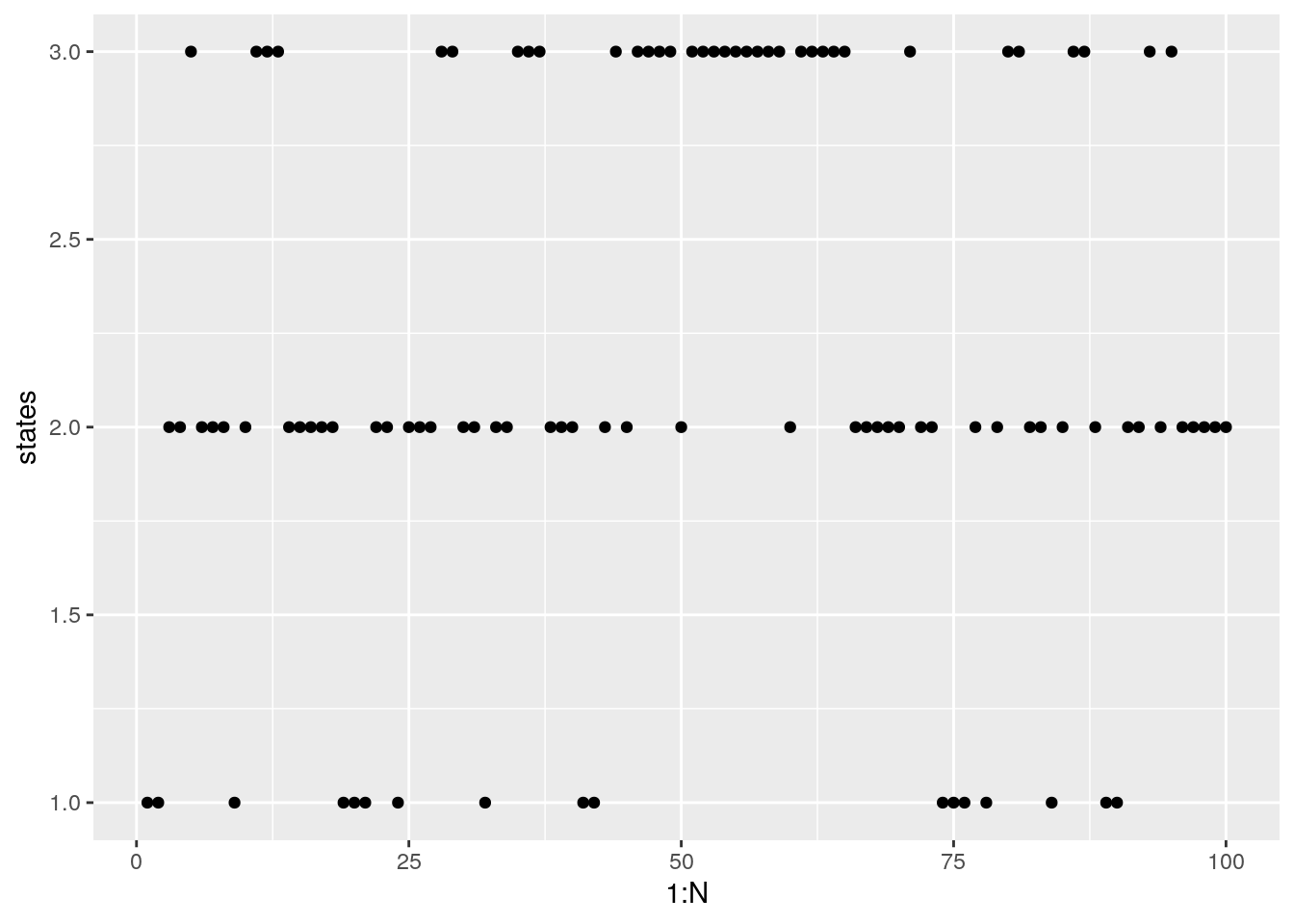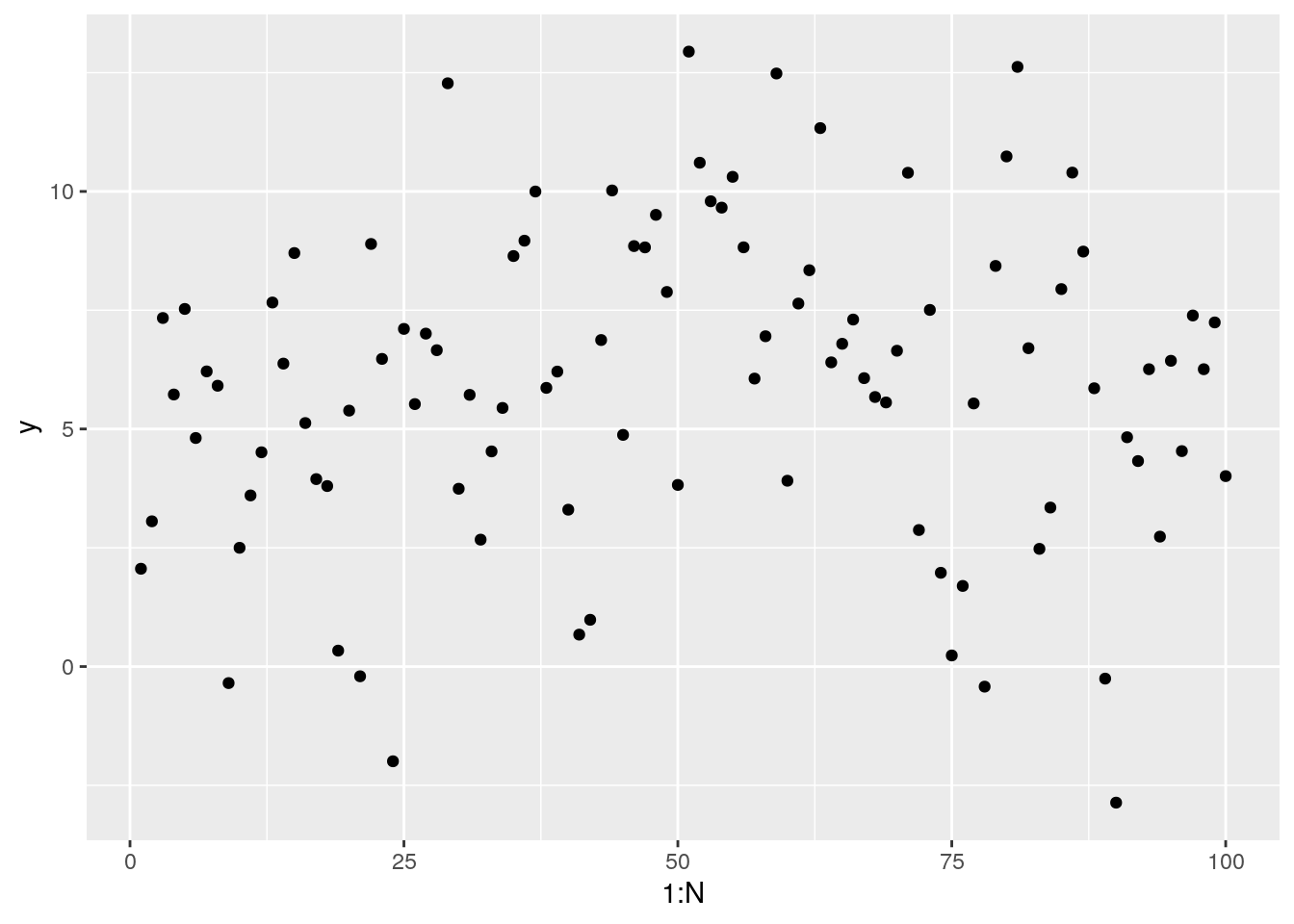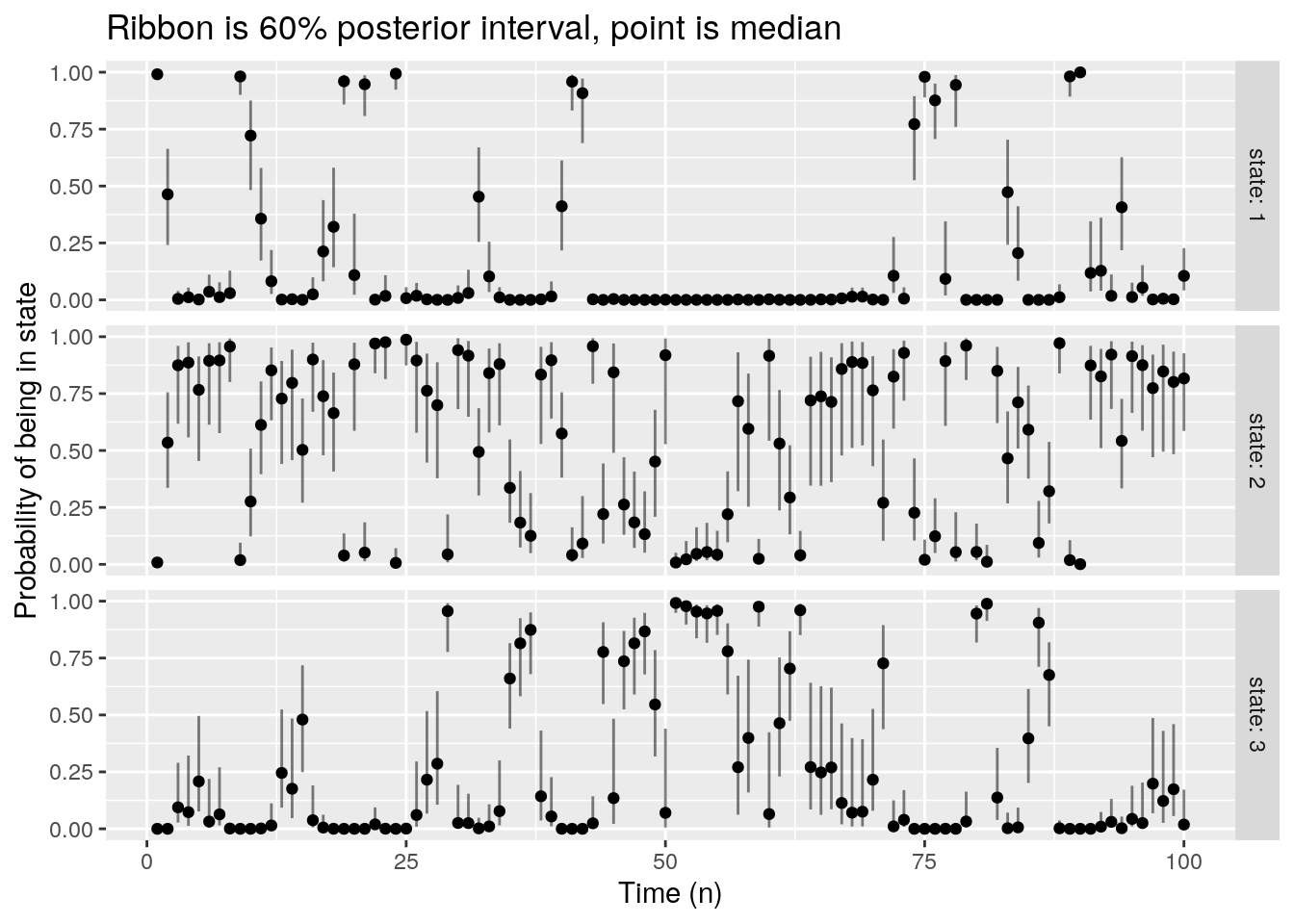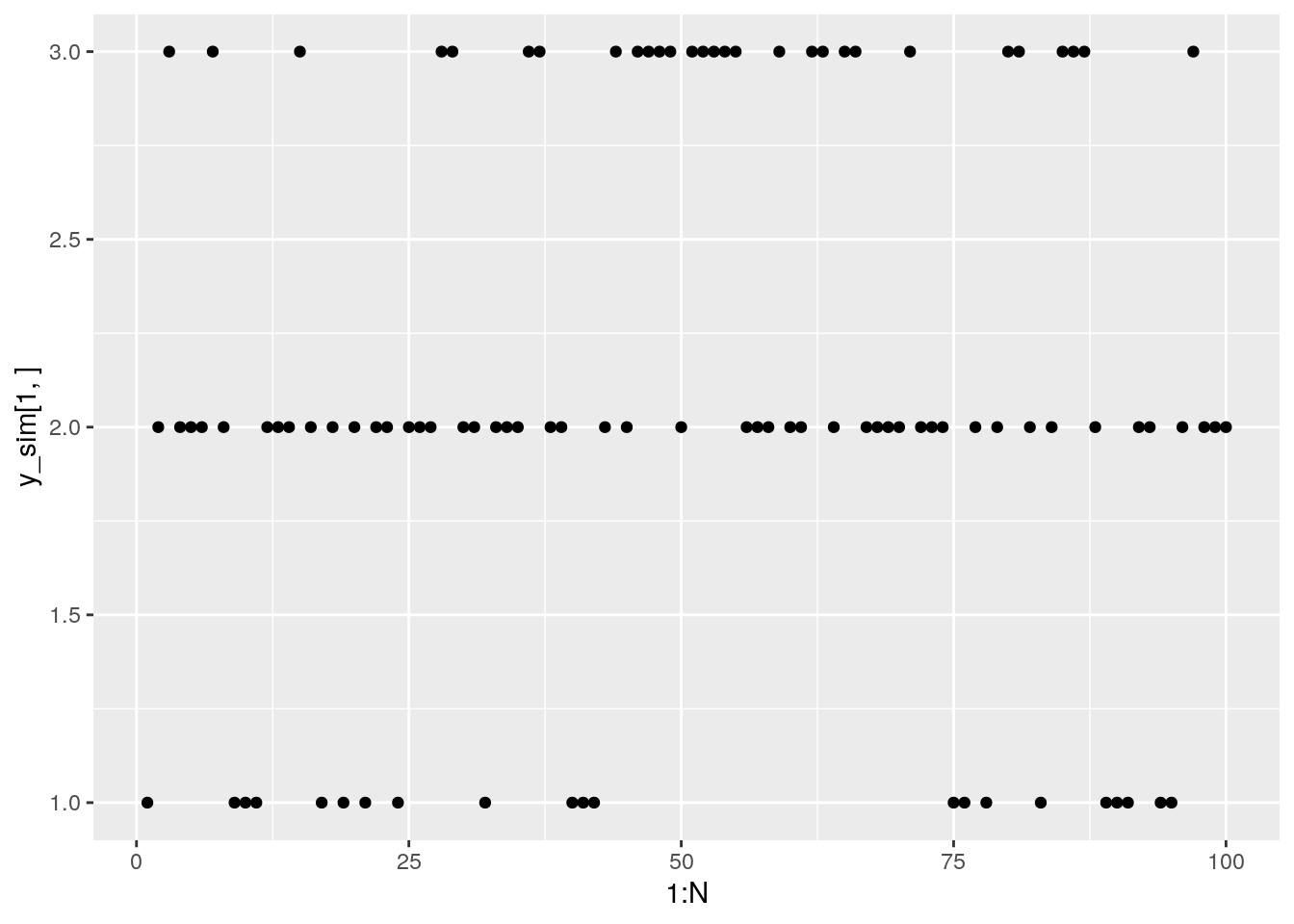## Introduction

CmdStan 2.24 introduced a new interface for fitting Hidden Markov models (HMMs) in Stan. This document is intended to provide an example use of this interface.

HMMs model a process where a system probabilistically switches between $$K$$ states over a sequence of $$N$$ points in time. It is assumed that the exact state of the system is unknown and must be inferred at each state.

HMMs are characterized in terms of the transition matrix $$\Gamma_{ij}$$ (each element being the probability of transitioning from state $$i$$ to state $$j$$ between measurements), the types of measurements made on the system (the system may emit continuous or discrete measurements), and the initial state of the system. Currently the HMM interface in Stan only supports a constant transition matrix. Future versions will support a transition matrix for each state.

Any realization of an HMM’s hidden state is a sequence of $$N$$ integers in the range $$[1, K]$$, however, because of the structure of the HMM, it is not necessary to sample the hidden states to do inference on the transition probabilities, the parameters of the measurement model, or the estimates of the initial state. Posterior draws from the hidden states can be computed separately.

A more complete mathematical definition of the HMM model and function interface is given in the Hidden Markov Models section of the Function Reference Guide.

There are three functions

• hmm_marginal - The likelihood of an HMM with the hidden discrete states integrated out
• hmm_latent_rng - A function to generate posterior draws of the hidden state that are implicitly integrated out of the model when using hmm_marginal (this is different than sampling more states with a posterior draw of a transition matrix and initial state)
• hmm_hidden_state_prob - A function to compute the posterior distributions of the integrated out hidden states

This guide will demonstrate how to simulate HMM realizations in R, fit the data with hmm_marginal, produce estimates of the distributions of the hidden states using hmm_hidden_state_prob, and generate draws of the hidden state from the posterior with hmm_latent_rng.

### Generating HMM realizations

Simulating an HMM requires a set of states, the transition probabilities between those states, and an estimate of the initial states.

For illustrative purposes, assume a three state system with states 1, 2, 3.

The transitions happen as follows: 1. In state 1 there is a 50% chance of moving to state 2 and a 50% chance of staying in state 1 2. In state 2 there is a 25% chance of moving to state 1, a 25% change of moving to state 3, and a 50% chance of staying in state 2 3. In state 3 there is a 50% chance of moving to state 2 and a 50% chance of staying at state 3.

Assume that the system starts in state 1.

N = 100 # 100 measurements
K = 3   # 3 states
states = rep(1, N)
states = 1 # Start in state 1
for(n in 2:length(states)) {
if(states[n - 1] == 1)
states[n] = sample(c(1, 2), size = 1, prob = c(0.5, 0.5))
else if(states[n - 1] == 2)
states[n] = sample(c(1, 2, 3), size = 1, prob = c(0.25, 0.5, 0.25))
else if(states[n - 1] == 3)
states[n] = sample(c(2, 3), size = 1, prob = c(0.5, 0.5))
}

The trajectory can easily be visualized:

qplot(1:N, states)An HMM is useful when the hidden state is not measure directly (if the state was measured directly, it wouldn’t be hidden).

In this example the observations are assumed to be normally distributed with a state specific mean and some measurement error.

mus = c(1.0, 5.0, 9.0)
sigma = 2.0
y = rnorm(N, mus[states], sd = sigma)

Plotting the simulated measurements gives:

qplot(1:N, y)### Fitting the HMM

To make it clear how to use the HMM fit functions, the model here will fit the transition matrix, the initial state, and the parameters of the measurement model. It is not necessary to estimate all of these things in practice if some of them are known.

The data is the previously generated sequence of $$N$$ measurements:

data {
int N; // Number of observations
real y[N];
}

For the transition matrix, assume that it is known that states 1 and 3 are not directly connected. For $$K$$ states, estimating a full transition matrix means estimatng a matrix of $$O(K^2)$$ probabilities. Depending on the data available, this may not be possible and so it is important to take advantage of available modeling assumptions. The state means are estimated as an ordered vector to avoid mode-swap non-identifiabilities.

parameters {
// Rows of the transition matrix
simplex t1;
simplex t2;
simplex t3;

// Initial state
simplex rho;

// Parameters of measurement model
vector mu;
real<lower = 0.0> sigma;
}

The hmm_marginal function takes the transition matrix and initial state directly. In this case the transition matrix needs to be constructed from t1, t2, and t3 but that is relatively easy to build.

The measurement model, in contrast, is not passed directly to the HMM function.

Instead, a $$K \times N$$ matrix log_omega of log likelihoods is passed in. The $$(k, n)$$ entry of this matrix is the log likelihood of the $$nth$$ measurement given the system at time $$n$$ is actually in state $$k$$. For the generative model above, these are log normals evaluated at the three different means.

transformed parameters {
matrix[3, 3] gamma = rep_matrix(0, 3, 3);
matrix[3, N] log_omega;

// Build the transition matrix
gamma[1, 1:2] = t1;
gamma[2, ] = t2;
gamma[3, 2:3] = t3;

// Compute the log likelihoods in each possible state
for(n in 1:N) {
// The observation model could change with n, or vary in a number of
//  different ways (which is why log_omega is passed in as an argument)
log_omega[1, n] = normal_lpdf(y[n] | mu, sigma);
log_omega[2, n] = normal_lpdf(y[n] | mu, sigma);
log_omega[3, n] = normal_lpdf(y[n] | mu, sigma);
}
}

With all that in place, the only thing left to do is add priors and increment the log density:

model {
mu ~ normal(0, 1);
sigma ~ normal(0, 1);

target += hmm_marginal(log_omega, Gamma, rho);
}

The complete model is available on Github: hmm-example.stan.

model = cmdstan_model("hmm-example.stan")
fit = model$sample(data = list(N = N, y = y), parallel_chains = 4) The estimated group means match the known ones: fit$summary("mu")
## # A tibble: 3 x 10
##   variable  mean median    sd   mad     q5   q95  rhat ess_bulk ess_tail
##   <chr>    <dbl>  <dbl> <dbl> <dbl>  <dbl> <dbl> <dbl>    <dbl>    <dbl>
## 1 mu     1.34   1.23 1.03  0.905 -0.120  3.25  1.00    1101.    1451.
## 2 mu     5.83   5.90 0.794 0.533  4.48   6.93  1.01    1276.    1045.
## 3 mu     9.17   9.26 1.05  1.06   7.48  10.7   1.01     792.    1482.

The estimated initial conditions are not much more informative than the prior, but it is there:

fit$summary("rho") ## # A tibble: 3 x 10 ## variable mean median sd mad q5 q95 rhat ess_bulk ess_tail ## <chr> <dbl> <dbl> <dbl> <dbl> <dbl> <dbl> <dbl> <dbl> <dbl> ## 1 rho 0.844 0.866 0.103 0.0966 0.645 0.971 1.00 2861. 2003. ## 2 rho 0.0795 0.0549 0.0768 0.0568 0.00381 0.239 1.00 2670. 1822. ## 3 rho 0.0766 0.0561 0.0716 0.0563 0.00401 0.218 1.00 3121. 2682. The transition probabilities from state 1 can be extracted: fit$summary("t1")
## # A tibble: 2 x 10
##   variable  mean median    sd   mad    q5   q95  rhat ess_bulk ess_tail
##   <chr>    <dbl>  <dbl> <dbl> <dbl> <dbl> <dbl> <dbl>    <dbl>    <dbl>
## 1 t1    0.403  0.396 0.169 0.166 0.127 0.693  1.00    2429.    1849.
## 2 t1    0.597  0.604 0.169 0.166 0.307 0.873  1.00    2429.    1849.

Similarly for state 2:

fit$summary("t2") ## # A tibble: 3 x 10 ## variable mean median sd mad q5 q95 rhat ess_bulk ess_tail ## <chr> <dbl> <dbl> <dbl> <dbl> <dbl> <dbl> <dbl> <dbl> <dbl> ## 1 t2 0.209 0.191 0.0992 0.0793 0.0864 0.400 1.00 1743. 1770. ## 2 t2 0.543 0.585 0.183 0.150 0.151 0.776 1.01 1579. 1355. ## 3 t2 0.248 0.219 0.136 0.107 0.0797 0.527 1.01 2133. 1777. And state 3: fit$summary("t3")
## # A tibble: 2 x 10
##   variable  mean median    sd   mad    q5   q95  rhat ess_bulk ess_tail
##   <chr>    <dbl>  <dbl> <dbl> <dbl> <dbl> <dbl> <dbl>    <dbl>    <dbl>
## 1 t3    0.444  0.437 0.187 0.183 0.155 0.782  1.00    1360.     939.
## 2 t3    0.556  0.563 0.187 0.183 0.218 0.845  1.00    1360.     939.

### State Probabilities

Even though the hidden states are integrated out, the distribution of hidden states at each time point can be computed with the function hmm_hidden_state_prob:

generated quantities {
matrix[3, N] hidden_probs = hmm_hidden_state_prob(log_omega, Gamma, rho);
}

These can be plotted:

hidden_probs_df = fit$draws() %>% as_draws_df %>% select(starts_with("hidden_probs")) %>% pivot_longer(everything(), names_to = c("state", "n"), names_transform = list(k = as.integer, n = as.integer), names_pattern = "hidden_probs\$([0-9]*),([0-9]*)\$", values_to = "hidden_probs") hidden_probs_df %>% group_by(state, n) %>% summarize(qh = quantile(hidden_probs, 0.8), m = median(hidden_probs), ql = quantile(hidden_probs, 0.2)) %>% ungroup() %>% ggplot() + geom_errorbar(aes(n, ymin = ql, ymax = qh, width = 0.0), alpha = 0.5) + geom_point(aes(n, m)) + facet_grid(state ~ ., labeller = "label_both") + ggtitle("Ribbon is 60% posterior interval, point is median") + ylab("Probability of being in state") + xlab("Time (n)") ## summarise() regrouping output by 'state' (override with .groups argument)If it is more convenient to work with draws of the hidden states at each time point (instead of the probabilities provided by hmm_hidden_state_prob), these can be generated with hmm_latent_rng: generated quantities { int[N] y_sim = hmm_latent_rng(log_omega, Gamma, rho) } Note that the probabilities from hmm_hidden_state_prob are the marginal probabilities of the hidden states, meaning they cannot be directly used to jointly sample hidden states. The posterior draws generated by hmm_latent_rng account for the correlation between hidden states. Note further these are draws of the hidden state that was integrated out. This is different than sampling new HMM realizations using posterior draws of the initial condition and the transition matrix. The draws of the hidden state can be plotted as well: y_sim = fit$draws() %>%
as_draws_df() %>%
select(starts_with("y_sim")) %>%
as.matrix

qplot(1:N, y_sim[1,])